Next: Locally projective nonlinear noise Up: Nonlinear noise reduction Previous: Nonlinear noise reduction

## Simple nonlinear noise reduction

The simplest nonlinear noise reduction algorithm we know of replaces the central coordinate of each embedding vector by the local average of this coordinate. This amounts to a locally constant approximation of the dynamics and is based on the assumption that the dynamics is continuous. The algorithm is described in , a similar approach is proposed in . In an unstable, for example chaotic, system, it is essential not to replace the first and last coordinates of the embedding vectors by local averages. Due to the instability, initial errors in these coordinates are magnified instead of being averaged out.

This noise reduction scheme is implemented quite easily. First an embedding has to be chosen. Except for extremely oversampled data, it is advantageous to choose a short time delay. The program lazy always uses unit delay. The embedding dimension m should be chosen somewhat higher than that required by the embedding theorems. Then for each embedding vector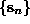, a neighborhood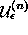is formed in phase space containing all points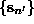such that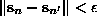. The radius of the neighborhoodsshould be taken large enough in order to cover the noise extent, but still smaller than a typical curvature radius. These conditions cannot always be fulfilled simultaneously, in which case one has to repeat the process with several choices and carefully evaluate the results. If the noise level is substantially smaller than the typical radius of curvature, neighborhoods of radius about 2-3 times the noise level gave the best results with artificial data. For each embedding vector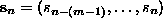(the delay time has been set to unity), a corrected middle coordinate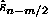is computed by averaging over the neighborhood: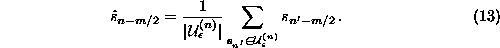After one complete sweep through the time series, all measurements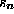are replaced by the corrected values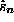. Of course, for the first and last (m-1)/2 points (if m is odd), no correction is available. The average correction can be taken as a new neighborhood radius for the next iteration. Note that the neighborhood of each point at least contains the point itself. If that is the only member, the average Eq.() is simply the uncorrected measurement and no change is made. Thus one can safely perform multiple iterations with decreasing values ofuntil no further change is made.

Let us illustrate the use of this scheme with an example, a recording of the air flow through the nose of a human as an indicator of breath activity. (The data is part of data set B of the Santa Fe time series contest held in 1991/92 , see Rigney et al.  for a description.) The result of simple nonlinear noise reduction is shown in Fig..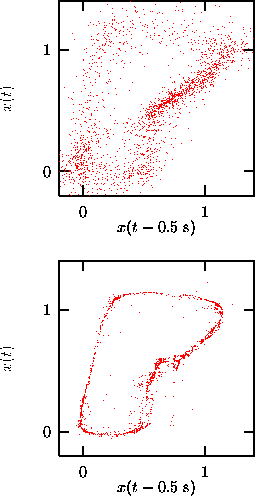Figure:   Simple nonlinear noise reduction of human breath rate data. Three iterations have been carried out, staring with neighborhoods of size 0.4 units. Embeddings in 7 dimensions at unit delay have been used. Arguably, the resulting series (lower panel) is less noisy. However, in Sec.we will show evidence that the noise is not just additive and independent of the signal.Next: Locally projective nonlinear noise Up: Nonlinear noise reduction Previous: Nonlinear noise reduction

Thomas Schreiber
Wed Jan 6 15:38:27 CET 1999